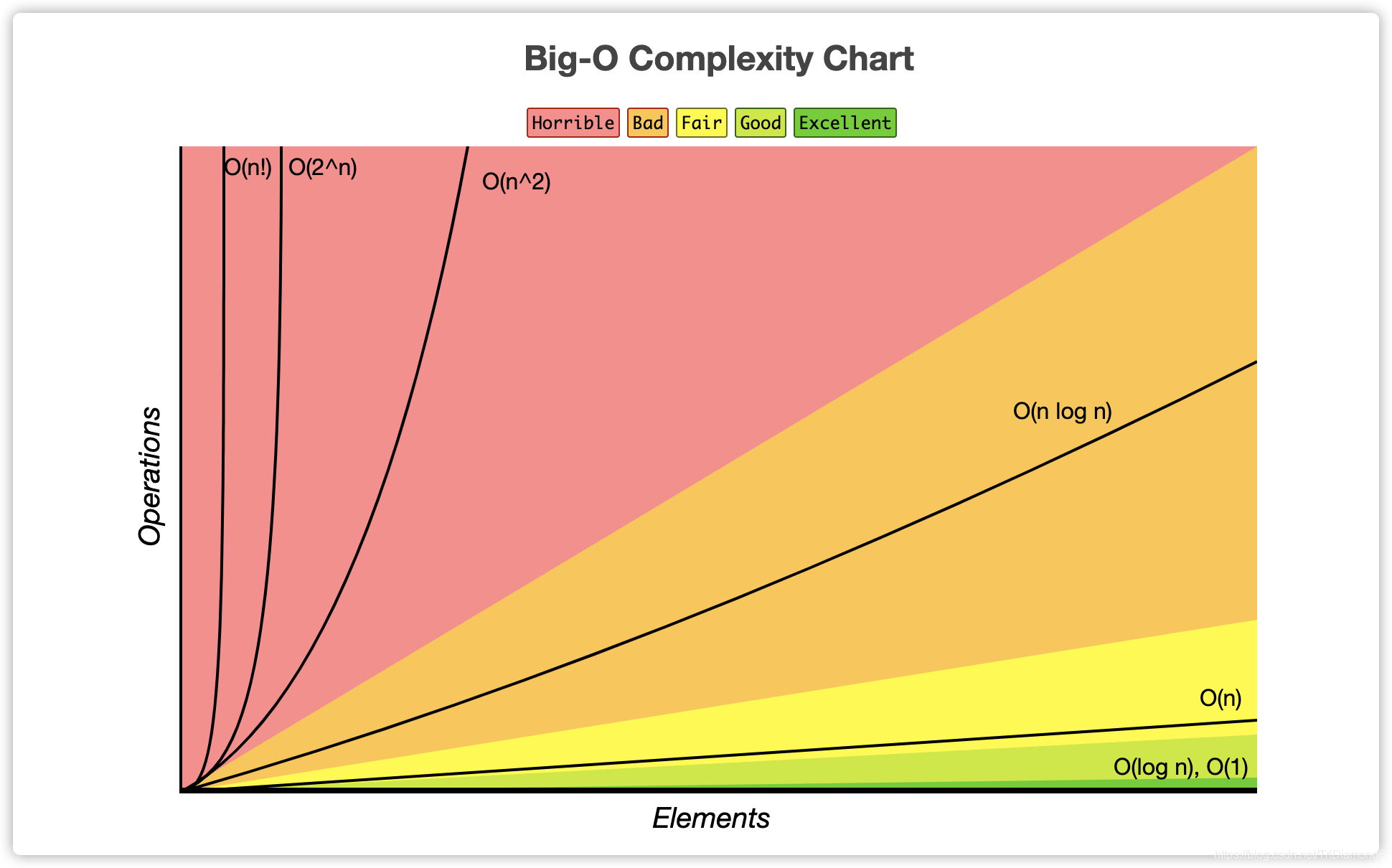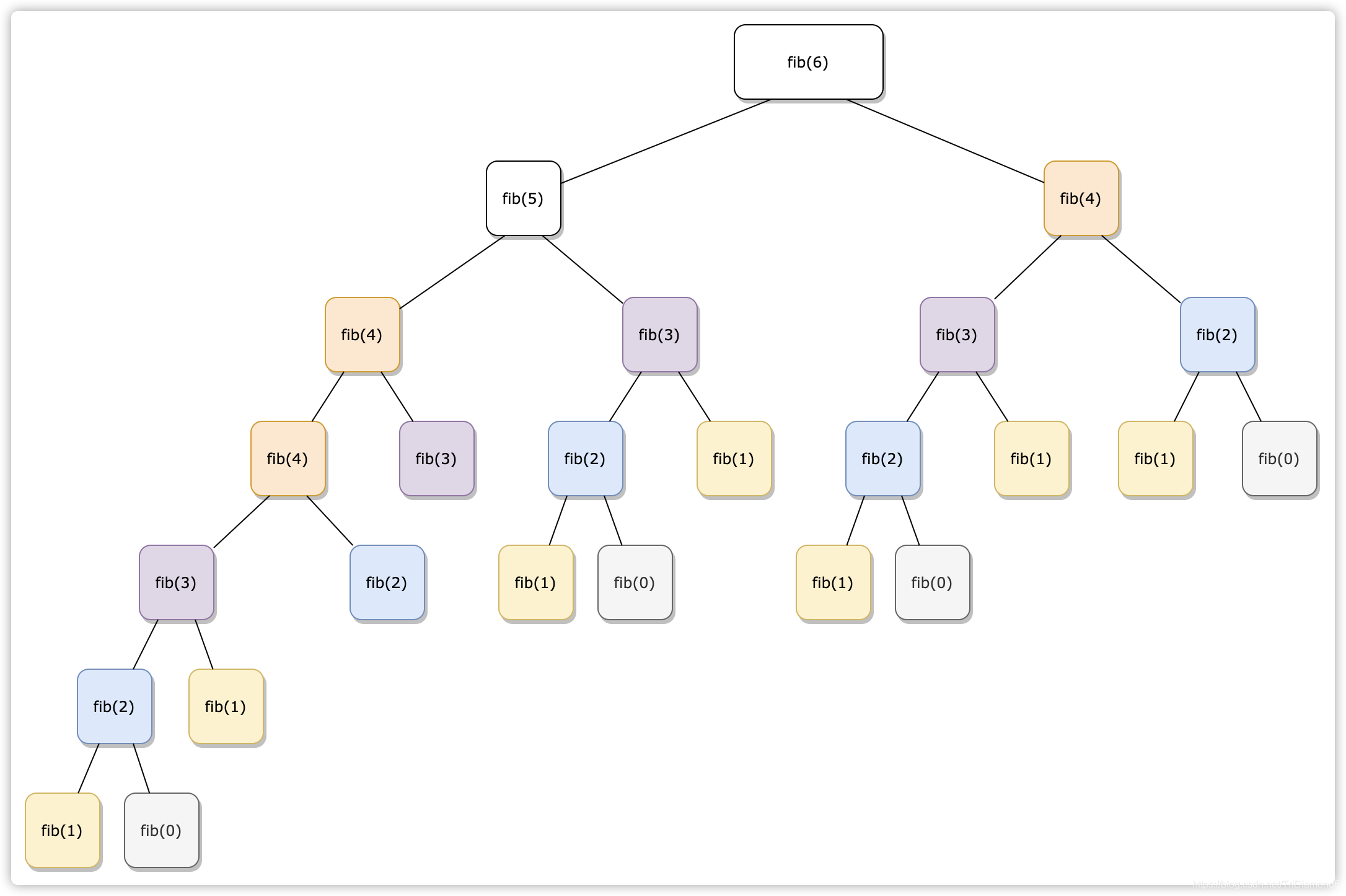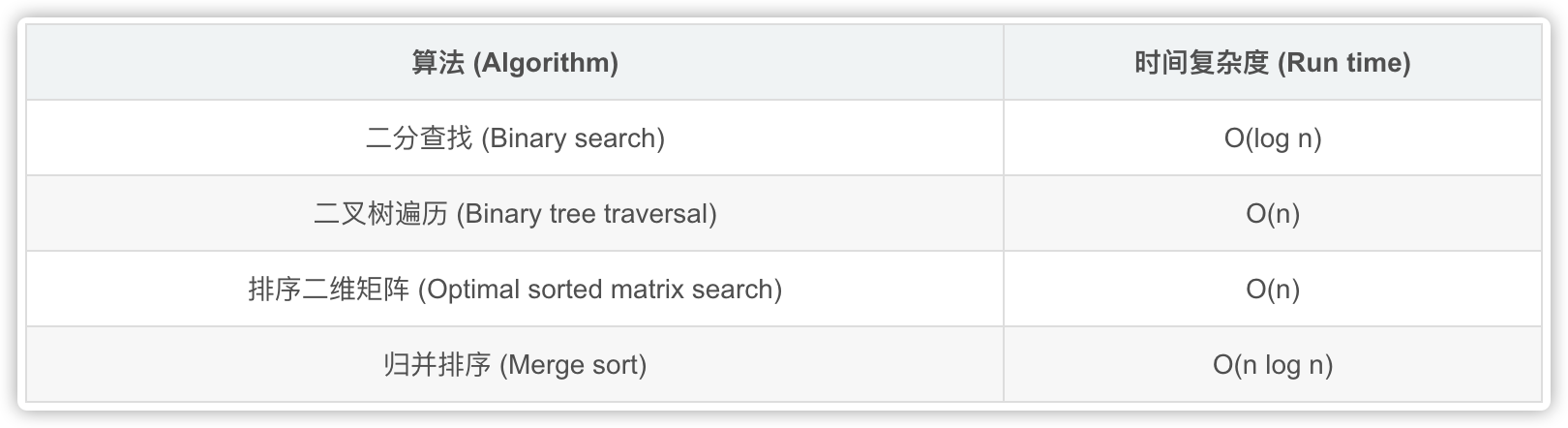# 【数据结构与算法】分析时间复杂度与空间复杂度﻿

﻿

# 前言

﻿

﻿

﻿

﻿

1. 弄懂编程的底层逻辑；

2. 在编程的过程中，拥有一个哆啦A梦一样百宝工具袋；

3. 在遇到性能问题的时候，有算法的思维逻辑和规则来解决问题；

4. 提高编程思维；

﻿

﻿﻿

# 复杂度指标 Big O Notation

﻿

• O (1): 常数复杂度 - Constant Complexity

• O (log n): 对数复杂度 - Logarithmic Complexity

• O (n): 线性复杂度 - Linear Complexity

• O (n^2): 平方复杂度 - N square Complexity

• O (2^n): 指数 - Exponential Growth

• O (n!): 阶乘 - Factorial

﻿

## 如何看时间复杂度

﻿

• 分析函数；

• 根据n的不同情况会运行多少次；

• 最后得出一个平均的运行次数的量级；

﻿

## Complexity 例子

﻿

O (1) - 常数复杂度

﻿

let n = 1000;console.log("Hello - your input is: " + n)

﻿

O (N) - 线性复杂度

﻿

for (let i = 1; i <= n; i++) {  console.log("Hello world - your input is: " + i)}

﻿

O (N^2)

﻿

for (let i = 1; i <= n; i++) {  for (let j = 1; j <= n; j++) {  	console.log("Hello world - your input is: " + i + " and " + j)  }}

﻿

﻿

for (let i = 1; i <= n; i++) {  console.log("Hello world - your i input is: " + i)}for (let j = 1; j <= n; j++) {  console.log("Hello world - your j input is: " + j)}

﻿

﻿

O(log(n))

﻿

for (let i = 1; i < n; i = i * 2) {  console.log("Hello world - your input is: " + i);}

﻿

O(k^n)

﻿

// Fibonacci递归function fib (n) {  if (n <= 2) return n;  return fib(n-1) + fib(n-2);}

﻿﻿

## 时间复杂度曲线

﻿

• y轴是Operations就是操作复杂度的指数；

• x轴是Elements就是n我们的循环次数 ；

• 这里我们可以看到在n比较小的时候，复杂度是相对稳定的；

• 但是当n越来越大时，Big-O复杂度就会急速飙升；

﻿

﻿

• 在编写程序的时候一定要注意到它的时间和空间复杂度，这样编写的时候就能预测出这段代码的性能级别；

• 用最简洁的时间和空间复杂度完成这段程序；

• 这样就是最顶尖的职业编程选手了；

• 因为复杂度越高，程序损耗的时间（处理时间）和资源（内存）就越大；

﻿

### 降低时间和空间复杂度

﻿

﻿

﻿

﻿

let sum = 0for (let i = 1; i < n; i++) {  sum += i}console.log(sum)

﻿

﻿

let sum = n * (n + 1) / 2console.log(sum)

﻿

1. 在做题或者面试的时候先确认题目，确保一切的条件和题目的理解无误；

2. 想出所有可能的解决方案；

3. 同时比较每个方法的时间和空间复杂度；

4. 接下来找出最优的解决方案（时间最快，内存使用最少）

﻿

### 判断时间和空间复杂度

﻿

﻿

﻿

﻿

function fib(n) {  if (n <= 2) return n  return fib(n - 1) + fib(n - 2)}

﻿

• 这个fib斐波那契函数中是一个递归

• 每一次传入一个n值时，都会循环递归fib方法来一层一层往下计算；

• 最后到达n小于2，返回最后的n值；

﻿

﻿

• 要推断出这个程序的复杂度，首先我们要知道具体在这个函数中程序做了什么；

• 我们距离现在传入n6，那就是运行fib(6)

• 这个时候6被传入这个方法，然后返回的就是fib(5)+fib(4)，这时fib(5)fib(4)就会再进入fib函数，这里就分开了两个分支了。以此类推我们就会出现以下一个树状过程：

﻿﻿

• 通过上图展开来的树，我们可以看到每一层是上一层的2倍：fib(6)展开为fib(5)+fib(4)，然后fib(5)fib(4)又展开了两个。

• 所以fibonacci的执行次数就是一个指数级 - O(2^n)

• 这里我们也可以看到fib(3)fib(4)等等，都被重复计算了多次，所以这个计算的复杂度高达2的6次方

• 所以在做题和面试的时候就不要运用上面的代码实例，我们要加入缓存机制，缓存重复计算的结果或者用一个循环来写，从而降低这个程序的复杂度。

﻿﻿

# 主定理 Master Theorem

﻿

﻿﻿

# 常见面试题

﻿

• 二叉树遍历中的前序、中序、后序：时间复杂度是多少？

+ 时间复杂度是 O(n)，无论是前序、中序或者后序每一个节点都会访问一次，并且仅访问一次；

+ 所以就是二叉树的节点总数，也就是O(n)的线性时间复杂度；

• 图的遍历：时间复杂度是多少？

+ 时间复杂也是O(n), 这里的n就是图里面的节点总数；

• 搜索算法：DFS、BFS时间复杂度是多少？

+ DFS是深度优先，BFS是广度优先算法。

+ 不管是深度优先还是广度优先，因为访问的节点只访问一次，所以时间复杂度也是O(n)的。（n指的是搜索空间里面的节点总数）

• 二分查找：时间复杂度是多少？

+ 答案是O(log n)

﻿﻿

# 总结

﻿

• 程序复杂度：Big O Notation

• O (1)，*O(log n)*， O(n)，*O(n^2)*, ... 等等，越复杂程序性能越差；

• 分析复杂度法则：分析代码的逻辑，找到程序中运行的次数；

• 降低程序时间和空间复杂度可以提升代码的质量，同时优化程序的性能；

• 主定理：

• 所有的分治或者*递归函数*都可以通过主定理来分析出它的时间复杂度

• 常见面试题：

• 二叉树遍历中的前序、中序、后序：时间复杂度是多少？ - O(n)

• 图的遍历：时间复杂度是多少？ - O(n)

• 搜索算法：DFS、BFS时间复杂度是多少？ - O(n)

• 二分查找：时间复杂度是多少？ - O(log n)

﻿

﻿

﻿## 评论